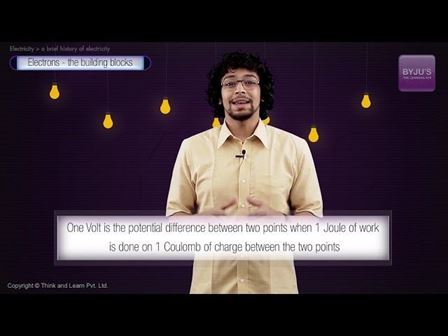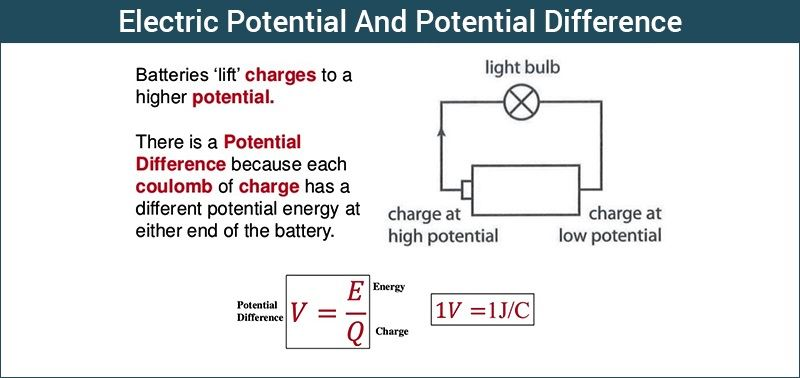# Electric Potential And Potential Difference

Electrical potential difference is the amount of work done to carrying a unit charge from one point to another in an electric field. We can also define potential difference as the difference in the electric potential of the two charged bodies.

 Common symbols: V, ∆V, U, ∆U Dimension: M L2 T−3 I−1 Derivations from other quantities: Voltage = Energy / charge SI unit: VoltIn a hydraulic circuit, we observe that water does not flow from one point to another if both the points are at the same height from the ground. Here, if we connect one end of the tube to a tank placed at a higher level from the ground, water starts to flow from the tank at higher level to the point at a lower level. Similarly, charge does not flow from one point to another until there is a potential difference between the two points. But what is electric potential?## What is Electric Potential?

An object rose against gravity, gains some gravitational potential energy proportional to the work done required to raise the object against the gravitational pull. Similarly, in an electric field, when an object is moved against the electric field it gains some amount of energy which is defined as the electric potential energy.

The magnitude of this energy depends on the amount of work done on the object in moving the object from one point to another against the electric field.  Electric potential energy is defined as the total amount of work done by an external agent in moving a charge from an arbitrarily chosen reference point, which we usually take as infinity ( as it is assumed that electrical potential of a charge at infinity is zero) to that position without any acceleration. For any charge,

Electric potential energy is defined as the total amount of work done by an external agent in moving a charge from an arbitrarily chosen reference point, which we usually take as infinity ( as it is assumed that electrical potential of a charge at infinity is zero) to that position without any acceleration. For any charge, electric potential is obtained by dividing the potential energy by the quantity of charge.

## What is Electric Potential Difference?

In an electrical circuit, potential between two points is defined as the amount of work done by an external agent in moving a unit charge from one point to another.

Mathematically we can say that,

 $E = \frac{W}{Q}$

Where,

• E = electrical potential difference between two points
• W = Work done in moving a change from one point to another
• Q = the quantity of charge in coulombs

The potential difference is measured by an instrument called voltmeter. The two terminals of a voltmeter are always connected parallel across the points whose potential is to be measured. Stay tuned with BYJU’S to learn more interesting physics topics with the help of interactive video lessons.

#### Practise This QuestionIn the given picture the Ring A at the bottom is positively charged. Another Ring B is brought and placed over it. Ring B hovers at a certain height above A. What could be the charge on Ring B.